Courses

# Parallel Plate Capacitors Dielectric Slab and Conducting Slab Class 12 Notes | EduRev

## JEE : Parallel Plate Capacitors Dielectric Slab and Conducting Slab Class 12 Notes | EduRev

The document Parallel Plate Capacitors Dielectric Slab and Conducting Slab Class 12 Notes | EduRev is a part of the JEE Course Physics Class 12.
All you need of JEE at this link: JEE

Dielectric Polarisation
When an external electric field is applied to a dielectric material, its behavior can be determined and is known as Dielectric Polarization that can be understood by the displacement of charges(positive and negative) when an electric field is applied

The main task of the dielectric polarization is to relate macroscopic properties to microscopic properties. Where macroscopic property can be dielectric constant to polarizability

Polarization occurs through the action of an electric field or other external factors, such as mechanical stress in the case of piezoelectric crystals(piezoelectric crystals are those solid materials which accumulates electric charge within them). Dielectric polarization can also arise spontaneously in pyroelectric crystals, particularly in ferroelectrics (Ferroelectricity is a property of certain materials that have a spontaneous electric polarization that can be reversed by the application of an external electric field).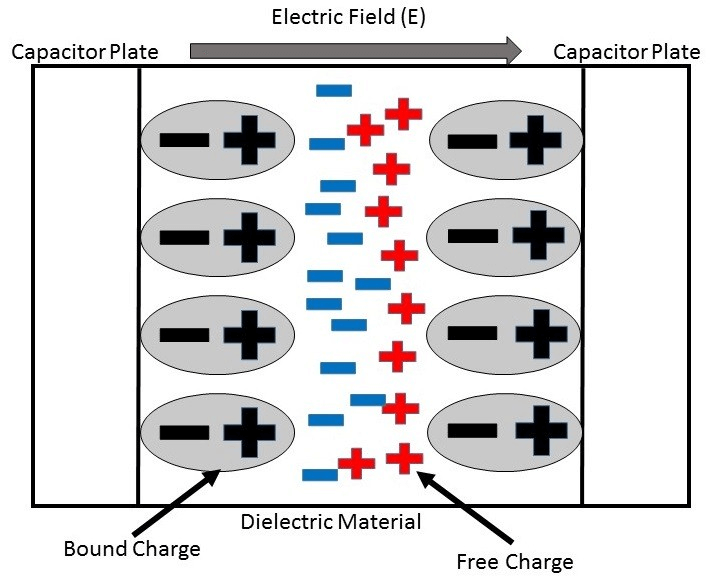Fig: Dielectric Polarisation

Inserting dielectric in Capacitor
When a dielectric material is introduced between the plates And when a dielectric material is placed between the plates of parallel plate capacitor then due to polarization of charges on either side of the dielectric, it produces an electric field of its own which acts in a direction opposite to that of the field due to the source.
This makes the net electric flux zero. This effect results in capacitance.

Effect of dielectric on plates when voltage or charge is held constant

Inserting a dielectric between the plates of capacitor while either the voltage or charge is held constant has the same effect that is the ratio of charge to voltage increases.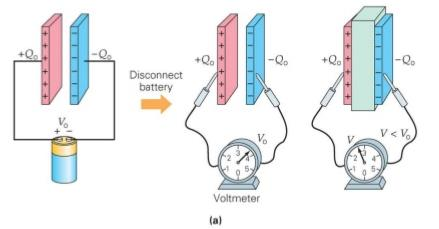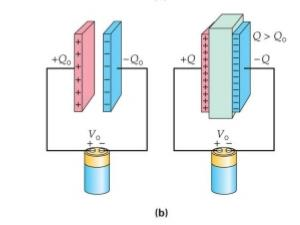Fig:

Effect of dielectric on plates when voltage or the charge is held constant

What does a dielectric do to a capacitor?
Dielectrics are often called Insulators when the insulator are introduced between the two plates of capacitor.
The side of the insulator closest to the positive plate will be negative, while the side closest to the negative plate will be positive.

The net field inside the capacitor and the potential difference across the capacitor is decreased when the electric field from the charge of capacitor plates are cancelled by the electric field from the polarized dielectric.More charge is needed by the capacitor to return to its original potential difference.
The net effect of the dielectric is to increase the amount of charge a capacitor can store for a given potential difference. The whole point of using a capacitor is to store charge, so coming up with a way to store more charge for the same amount of effort is a good thing.

Adding dielectric to a capacitor increases the capacitance by a factor of K and,
• Charge on the capacitor will increase when the voltage is held constant and
• If the capacitor is disconnected that is the charge is held constant the potential across the capacitor will decrease.

Capacitance of Parallel Plate Capacitor
The capacitance of parallel plate capacitor depends upon:
• The distance d between two plates
• The area A of medium between the plates
• According to the gauss law, the electric field can be written as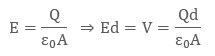Since we know that the capacitance is defined as V = Q/C, so capacitance can be rewritten as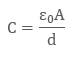When the plates are placed very close and the area of plates are large we get the maximum capacitance.

Capacitance of Parallel Plate Capacitor when dielectric slab is placed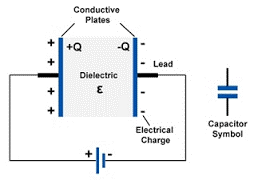Fig: dielectric placed between two electrodes

On the two plates, the microscopic dipole moment of the material will shield the charges. Thus, will alter the effect of dielectric material which is inserted between the two plates Materials have a permeability which is given by the relative permeability K
The capacitance is thus given by: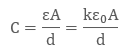Capacitance of a parallel plate capacitor can be increased by introducing dielectric between the plates as the dielectric have permeability k, which is greater than 1. K is also sometimes known as Dielectric Constant.

Capacitance in the presence of Dielectric
1. When dielectric is completely filled between the plates
When the area between the two plates is filled completely with dielectric then of relative permeability er,
C0 = C x er
The presence of dielectric will reduce the electric intensity by er times
Thus the capacitance is increased by er times.
2. When dielectric is partially filled between the plates
When in parallel plate capacitor the area between the who plates are partially filled with air and partially with other substance its Capacitance can be calculate.
Let there exist a parallel plate capacitor in which medium between the parallel plates is mainly the air and partially other substance.

Offer running on EduRev: Apply code STAYHOME200 to get INR 200 off on our premium plan EduRev Infinity!

,

,

,

,

,

,

,

,

,

,

,

,

,

,

,

,

,

,

,

,

,

;